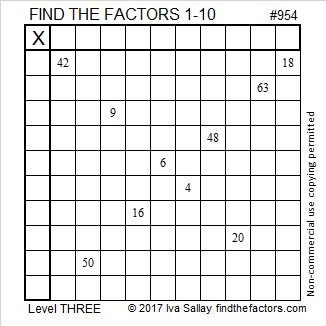# 954 and Level 3

The ten clues in this puzzle are all that is needed to solve the puzzle and then make it a complete multiplication table. Seriously, ten clues! Yes, the factors and the products will not be in their usual places, but the completed puzzle will still be a legitimate multiplication table. Can YOU make it work?Print the puzzles or type the solution in this excel file: 10-factors-951-958

954 is the sum of ten consecutive prime numbers:
73 + 79 + 83 + 89 + 97 + 101 + 103 + 107 + 109 + 113 = 954

27² +  15² = 954 so 954 is the hypotenuse of a Pythagorean triple:
504-810-954 which is 18 times (28-45-53)

954 is a palindrome in two consecutive bases:
676 in BASE 12 because 6(144) + 7(12) + 6(1) = 954
585 in BASE 13 because 5(169) + 8(13) + 5(1) = 954

• 954 is a composite number.
• Prime factorization: 954 = 2 × 3 × 3 × 53, which can be written 954 = 2 × 3² × 53
• The exponents in the prime factorization are 2, 1, and 1. Adding one to each and multiplying we get (1 + 1)(2 + 1)(1 + 1) = 2 × 3 × 2 = 12. Therefore 954 has exactly 12 factors.
• Factors of 954: 1, 2, 3, 6, 9, 18, 53, 106, 159, 318, 477, 954
• Factor pairs: 954 = 1 × 954, 2 × 477, 3 × 318, 6 × 159, 9 × 106, or 18 × 53,
• Taking the factor pair with the largest square number factor, we get √954 = (√9)(√106) = 3√106 ≈ 30.88689This site uses Akismet to reduce spam. Learn how your comment data is processed.